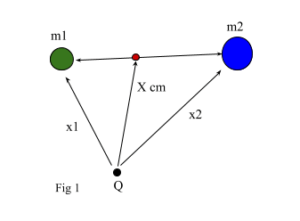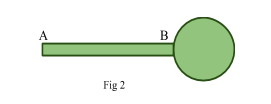# Centre of mass

Contents

## Summary

• The centre of mass of a body or a system of particles is defined as a single point at which the whole mass of the body or system is imagined to be concentrated and all the applied forces acts at that point.
• In other words the concept of the center of mass is as that of an average of the masses factored by their distances from a reference point.
• Formula to calculate the position of the center of mass with respect to a reference point is given as:${ X }_{ cm }\quad =\quad \frac { { m }_{ 1 }{ x }_{ 1 }\quad +\quad { m }_{ 2 }{ x }_{ 2 } }{ { m }_{ 1 }{ \quad +\quad m }_{ 2 } }$

Centre of mass is the point at which the distribution of mass is equal in all directions, and does not depend on gravitational field. The centre of mass of a body or a system of particles is defined as a single point at which the whole mass of the body or system is imagined to be concentrated and all the applied forces acts at that point. The motion of a body can be described as the motion of its centre of mass.

The centre of mass , has translational motion under the influence of forces. If a single force acts on a body and the line of action of the force passes through the centre of mass, the body will have linear acceleration but possess no angular acceleration. We can further describe this concept of the center of mass as that of an average of the masses factored by their distances from a reference point.

Consider a system formed by two point particles of masses${ m }_{ 1 }$  and${ m }_{ 2 }$  located at positions${ x }_{ 1 }$  and${ x }_{ 2 }$  measured with respect to point Q as shown in the figure 1 below. The position of the center of mass with respect to the coordinate system with origin at point Q is obtained as:${ X }_{ cm }\quad =\quad \frac { { m }_{ 1 }{ x }_{ 1 }\quad +\quad { m }_{ 2 }{ x }_{ 2 } }{ { m }_{ 1 }{ \quad +\quad m }_{ 2 } }$#### Example #1

Q. A metallic sphere of mass 1 Kg and radius 5 cm is welded to the end B of a thin rod AB of length 50 cm and mass 0.5 Kg as shown in Fig 2. Find the distance “x” such that this rod with the sphere will balance horizontally on a knife edge placed at a distance “x” from the end A of the rod.Solution:

Firstly we write the data that is provided in the question above${ m }_{ 1 }\quad =\quad 1\quad kg$${ x }_{ 1 }\quad =\quad 5\quad +\quad 50\quad =\quad 55\quad cm$${ m }_{ 2 }\quad =\quad 0.5\quad kg$${ x }_{ 2 }\quad =\quad \frac { 50 }{ 2 } \quad =\quad 25\quad cm$

We then use the formula below to calculate the distance “x”;${ X }_{ cm }\quad =\quad \frac { (1)(55)\quad +\quad (0.5)(25) }{ 1\quad +\quad \quad 0.8 }$${ X }_{ cm }\quad =\quad 45\quad cm$         Ans

#### Example #2

Q. An object weighing 120 N is set on a rigid beam of negligible mass at a distance of 3 m from a pivot, as shown below in Fig 3. A vertical force is to be applied to the other end of the beam a distance of 4m from the pivot to keep the beam at rest and horizontal. What is the magnitude F of the force required?Solution:

Firstly we write the data that is provided in the question above;${ m }_{ 1 }\quad =\quad 120\quad N$${ x }_{ 1 }\quad =\quad 3\quad m$${ m }_{ 2 }\quad =\quad F\quad N$${ x }_{ 2 }\quad =\quad 4\quad m$

Here reference point X is considered to be 0 m.

We then use the formula below to calculate the magnitude of force F:${ X }_{ cm }\quad =\quad \frac { { m }_{ 1 }{ x }_{ 1 }\quad +\quad { m }_{ 2 }{ x }_{ 2 } }{ { m }_{ 1 }{ \quad +\quad m }_{ 2 } }$${ X }_{ cm }\quad =\quad \frac { (120)(3)\quad +\quad (F)(4) }{ 120\quad +\quad F }$$4F\quad =\quad 360$$F\quad =\quad 90\quad N$         Ans